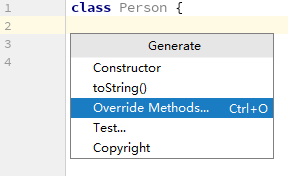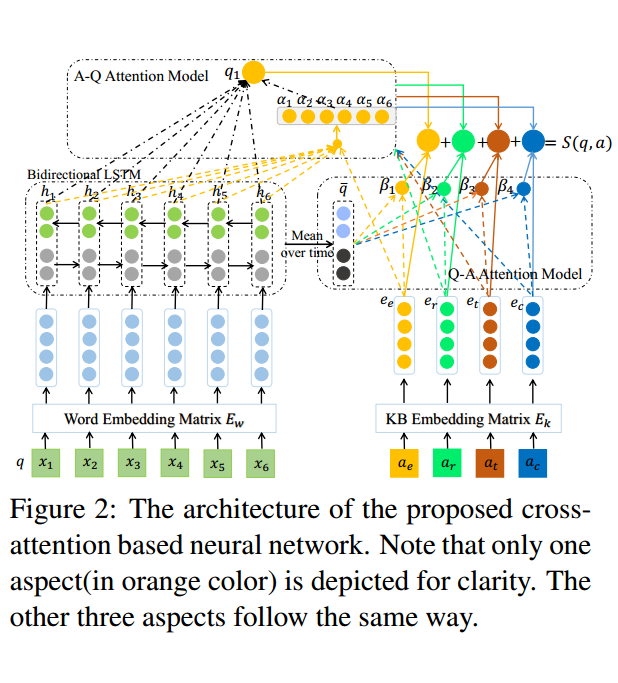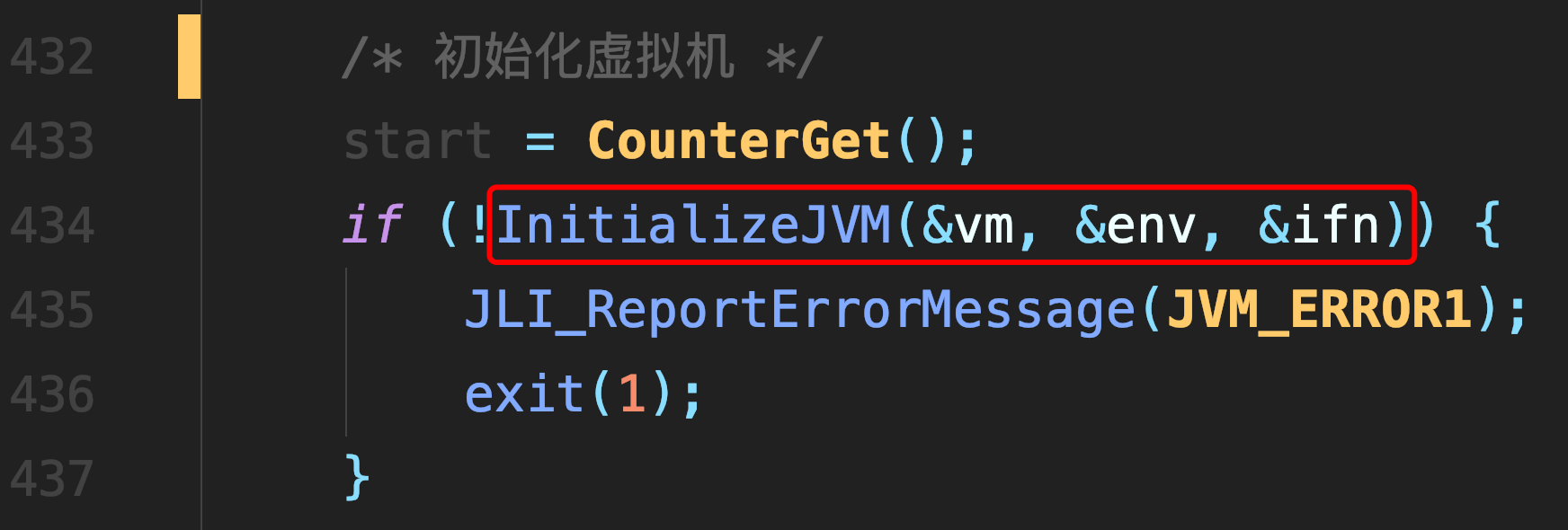# [转载] \$CF669A\$ 题解

``#include<bits/stdc++.h> using namespace std; inline int read() { int ret=0,f=1; char ch=getchar(); while(ch>'9'||ch<'0') { if(ch=='-') f=-1; ch=getchar(); } while(ch>='0'&&ch<='9') { ret=(ret<<1)+(ret<<3)+ch-'0'; ch=getchar(); } return ret*f; } int n; int dfs(int n,int step,int mi) { if(n<=2) return step+1; if(n>2) { if(mi==1) dfs(n-1,step+1,2); else dfs(n-2,step+1,1); } } int main() { n=read(); if(n<=2) printf("1\n"); else printf("%d\n",dfs(n,0,1)); return 0; }``

##### 热门推荐
•浏览(654)
•浏览(563)
•浏览(547)
•浏览(480)
•浏览(424)
•浏览(419)
•浏览(381)
•浏览(366)
•浏览(362)
•浏览(344)